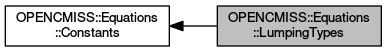OpenCMISS-Iron FORTRAN API Documentation
OPENCMISS::Equations::LumpingTypes

Equations lumping types. More...

Collaboration diagram for OPENCMISS::Equations::LumpingTypes:Variables

integer(intg), parameter, public opencmiss_iron::cmfe_equations_unlumped_matrices = EQUATIONS_UNLUMPED_MATRICES
The equations matrices are not lumped. More...

integer(intg), parameter, public opencmiss_iron::cmfe_equations_lumped_matrices = EQUATIONS_LUMPED_MATRICES
The equations matrices are "mass" lumped. More...

Detailed Description

Equations lumping types.

OPENCMISS::Equations,OPENCMISS

Variable Documentation

 integer(intg), parameter, public opencmiss_iron::cmfe_equations_lumped_matrices = EQUATIONS_LUMPED_MATRICES

The equations matrices are "mass" lumped.